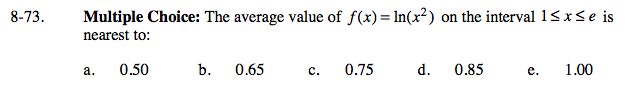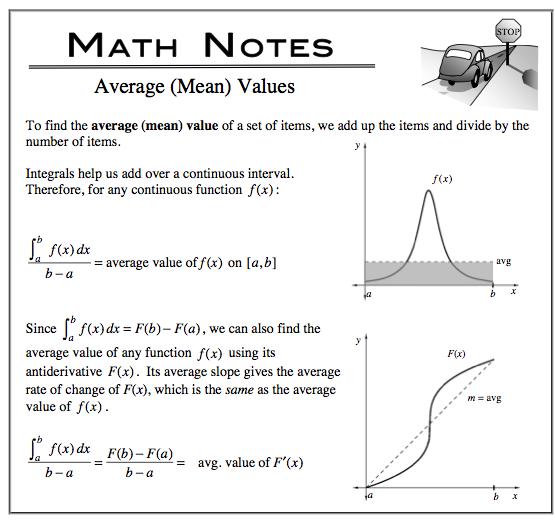### Home > CALC > Chapter 8 > Lesson 8.2.1 > Problem8-73

8-73.
1. Multiple Choice: The average value of f(x) = ln(x2) on the interval 1 ≤ x e is nearest to: Homework Help ✎

1. 0.50

2. 0.65

3. 0.75

4. 0.85

5. 1.00You are given f(x) and you want to find the average (mean) value of f(x). Will you integrate and divide, or will you find the slope of the secant line?